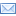SuperTeacherToolsArithmetic and Geometric Sequences Speed Match Review Game

This game has been played 2 times
The best time ever was Will at 264 seconds.
The best time today is at seconds.
How To Play:
Drag the squares in the gray area and drop them in the matching squares in the blue area below.
If you drop a square in the correct spot, it will disappear.
If you make a mistake, the square will return to its original spot.
Good luck!

Let's Play Speed Match!

d=7
1, -8, 64, -512, 4096
d=-11
a(1)=10 a(n)=a(n-1)-3
96, 48, 24, 12, 6
-166
a(1)=-2 a(n)=-5a(n-1)
-4, -16, -64, -256, -1024
a(n)=-6+(n-1)(5)
7, 13, 19, 25, 31
r=-4
r=3
a(1)=80 a(n)=1/2a(n-1)
r=1/3
280
a(1)=3 a(n)=2a(n-1)
a(n)=5+(n-1)(-4)
a(n)=8+(n-1)(-6)
a(1)=-4 a(n)=a(n-1)+2
80
139
a(n)=-7+(n-1)(-3)
-121
30, 21, 12, 3, -6
a(n)=19+(n-1)(9)
5, 15, 45, 135, 405, 1215
-6, -1, 4, 9, 14
3, 6, 12, 24, 48
5, 12, 19, 26, 33, 40
a(1)=-4 a(n)=4a(n-1)
10, 7, 4, 1, -2
19, 28, 37, 46, 55
a(1)=1 a(n)=-8a(n-1)
24, 13, 2, -9, -20, -31
a(1)=7 a(n)=a(n-1)+6
-6, -1, 4, 9, 14
a(1)=30 a(n)=a(n-1)-9
8, 2, -4, -10, -16
-4, -2, 0, 2, 4
-7, -4, -1, 2, 5
3, -12, 48, -192, 768, -3072
-7, -4, -1, 2, 5
5, 1, -3, -7, -11
-2, 10, -50, 250, -1250
5, 1, -3, -7, -11
1701, 567, 189, 63, 21, 7
8, 2, -4, -10, -16
80, 40, 20, 10, 5
19, 28, 37, 46, 55
a(1)=96 a(n)=0.5a(n-1)